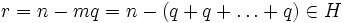# Tour:Every nontrivial subgroup of the group of integers is cyclic on its smallest element

(diff) ← Older revision | Latest revision (diff) | Newer revision → (diff)

This article adapts material from the main article: every nontrivial subgroup of the group of integers is cyclic on its smallest element

PREVIOUS: Equivalence of definitions of cyclic group| UP: Introduction four (beginners)|
General instructions for the tour | Pedagogical notes for the tour | Pedagogical notes for this part
WHAT YOU NEED TO DO:
• Read the statement and understand it thoroughly.
• Go through the proof very carefully. Make sure you understand all the steps of the proof, particularly the application of Euclidean division.

## Statement

Let$H$ be a subgroup of$\mathbb{Z}$, the group of integers under addition. Then, there are two possibilities:

•$H$ is the trivial subgroup, i.e.$H = \{ 0 \}$
•$H$ contains a smallest positive element, say$m$, and$H$ is the set of multiples of$m$. Thus,$H$ is an infinite cyclic group generated by$m$, and is isomorphic to$\mathbb{Z}$. We typically write$H = m\mathbb{Z}$.

## Proof

Given: A nontrivial subgroup$H$ of$\mathbb{Z}$, the group of integers under addition

To prove: There exists a smallest positive element$m$ in$H$, and$H = m \mathbb{Z}$, so$H$ is isomorphic to$\mathbb{Z}$

Proof: First, observe that if$H$ is nontrivial, then there exists a nonzero element in$H$. This element may be either positive or negative. However, since$H$ is a subgroup, it is closed under taking additive inverses, so even if the element originally picked was negative, we have found a positive number in$H$.

Thus, the set of positive numbers in$H$ is nonempty. Hence, there exists a smallest positive number in$H$. Call it$m$.

Clearly, all the integer multiples of$m$ are in$H$. We need to prove that every element in$H$ is a multiple of$m$.

By the Euclidean division algorithm, we can write:$n = mq + r$

where$q,r$ are integers and$0 \le r < m$. Since$n,q \in H$,$r = n - mq = n - (q + q + \dots + q) \in H$. Thus,$r$ is a nonnegative integer less than$m$ such that$r \in H$. By the minimality of$m$, we have$r = 0$, so$m | n$, as desired.

Thus,$H = m\mathbb{Z}$, or$H$ is the set of multiples of$m$.

An explicit isomorphism from$\mathbb{Z}$ to$H$ is given by the map sending an integer$x$ to the integer$mx$.